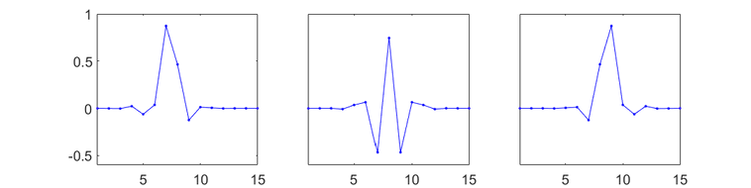##### New research: Wavelets and MERA

Recently a precise connection between discrete wavelets transformations (DWT's) and MERA tensor networks has been established. This connection has opened the possibility for using techniques developed in the context of tensor networks to design new wavelet transformations and also for the use of wavelet methods to  gain a better analytic understanding of MERA.

Here we demonstrate the use of a new wavelet design (TypeII, edge-centered symmetric wavelet from Fig.8(b) of this reference) for the purpose of image compression. We choose this wavelet design for the demonstration as it seems to be a good compromise between the accuracy (typically slightly improving over that of the wavelets used in JPEG2000 at the same compression ratio) and the computational cost of transformation (with only a slight cost increase over the CDF-9/7 transformation). In addition, these new wavelets have the advantage of being fully orthogonal, whereas the CDF wavelets are not.

This transformation is based on a depth-3 ternary unitary circuit (fully characterized by 3 rotation angles theta as given below), with a scaling function and two wavelet functions at each scale, as depicted below. During the transformation, two copies of this circuit are applied (one to the even sublattice and the other to the odd sublattice), whereupon symmetric / anti-symmetric scaling functions and wavelets are formed by taking the appropriate linear combinations from each circuit. The example code has the following features:

• Boundaries are treated using a symmetric extension

• Compatible with grey-scale or color images of any dimension

• Compression ratio can be specified

• The symmetric wavelets have 4 vanishing moments, while the anti-symmetric wavelets have 3 vanishing moments

###### Unitary gates:###### Scaling sequences:bL

bC

bR

###### Scaling functions and waveletssca

wav: mid

wav: high

##### Example usage: image compression###### Recovered image, PSNR 31.27